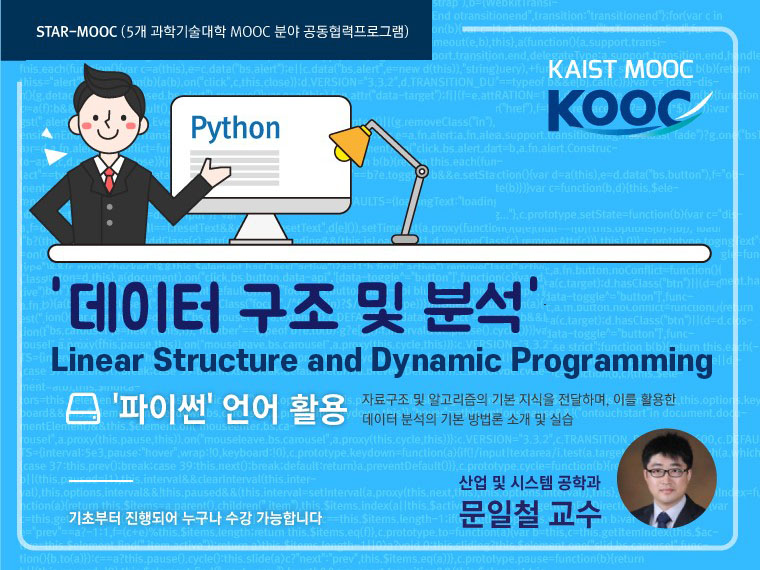KAIST 산업및시스템공학과 문일철
• Type MOOC course
• Period Always open
• Learning Time Study freely
• Course approval method Automatic approval
• Certificate Issue Online### Instructor Introduction

•KAIST 산업및시스템공학과 문일철

KAIST 산업 및 시스템 공학과 교수(2011.9~ )

### Lecture plan

★강의 수강
1. Chapter 0: 강좌 시작 전 꼭 확인해 주세요!
1. 수료기준, 게시판 등 강좌 학습 방법 소개
1. Orientation (Offline Orientation Video + Material)
2. Chapter 1 Python Overview
1. Programming and Execution Environment
1. Hello World in Python
1. Variable Statements and Operators
1. String
1. List, Tuple, Dictionary
1. Condition and Loop Satement
1. Function Statement
1. Assignment and Equivalence
1. Class and Instance
1. Module and Import
1. Quiz 1
3. Chapter 2 Object-oriented paradigm and software design
1. Design and Programming
1. UML notation 1
1. Encapsulation and Inheritance
1. Polymorphism and Abstract Class
1. UML notation 2
1. Structure and Relationship
1. Quiz 2
4. Chapter 3 Linked list, stack and queue
1. Abstract Data Types
1. Array
1. Stack
1. Queue
1. Quiz 3
5. Chapter 4 Recursion and dynamic programming
1. Recursions
1. Merge Sort and Problems in Recursions
1. Dynamic Programming 1 (Memoization)
1. Fibonacci Sequence in DP
1. Process of Assembly Line Scheduling
1. Assembly Line Scheduling in Recursion and DP
1. Quiz 4
6. Chapter 5 Application 1 : Simulation
1. Comprehend a problem
1. Introduction of modeling
1. Examples of simulation
1. Quiz 5
7. Chapter 6 Binary Search Tree
1. Tree as an Abstract Data Type and Structure
1. Terminologies of Tree Structure
1. Characteristics of Tree
1. Binary Search Tree and Implementation
1. Insert and Search Operation of Binary Search Tree
1. Delete Operation and Minimum & Maximum of Binary Search Tree
1. Tree Traversing
1. Quiz 6
8. Chapter 7 Algorithm Analysis
1. Factors of Program's Efficiency
1. Bubble Sort Algorithm
1. Importance of Efficiency
1. Definition of Algorithm Analysis and Examples
1. Big-Oh Notation
1. Growth Rate
1. Examples & Rules of Big-Oh Notation
1. Quiz 7
9. ★강의 수강 후 의견을 부탁드리겠습니다.★
1. 교수님 강의에 대한 별점을 매겨주세요. 여러분의 의견이 많은 도움이 됩니다:D
☆코드 실습 (설명강의 포함)
1. 데이터 구조 및 분석 : Linear Structure and Dynamic Programming - Setup
1. Anaconda & Pycharm Setup
2. Chapter 1 실습코드
1. Naive Bayes classifier - Conditional probability calculation
1. 오프라인 실습 수업 영상_Lecture 1 : Simple Sentiment Analysis
3. Chapter 2 실습코드
1. Naive Bayes classifier - Classifier with prior and likelihood
1. 오프라인 실습 수업 영상_Lecture 2 : Implementing Class with Diagram
4. Chapter 3 실습코드
1. Production line model - Stack and queue implementation
1. 오프라인 실습 수업 영상_Lecture 3 : Linked List, Stack and Queue
5. Chapter 4 실습코드
1. Production line model - Scheduling with dynamic programming
1. 오프라인 실습 수업 영상_Lecture 4 : Linked List, Stack and Queue (Continued)
6. Chapter 5 실습코드
1. Production line model - Probabilistic interrupt modeling
1. 오프라인 실습 수업 영상_Lecture 5 : Dynamic Programming
7. Chapter 6 실습코드
1. Decision Tree - Decision tree node implementation
1. 오프라인 실습 수업 영상_Lecture 6 : Tree
8. Chapter 7 실습코드
1. Decision Tree - Decision tree implementation
1. 오프라인 실습 수업 영상_Lecture 7: Binary Tree